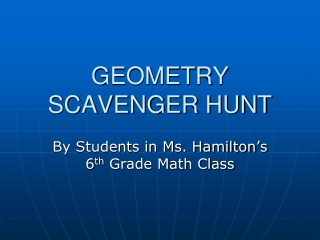DownloadDownload PresentationGEOMETRY SCAVENGER HUNT

# GEOMETRY SCAVENGER HUNT

Download Presentation## GEOMETRY SCAVENGER HUNT

- - - - - - - - - - - - - - - - - - - - - - - - - - - E N D - - - - - - - - - - - - - - - - - - - - - - - - - - -
##### Presentation Transcript

1. GEOMETRY SCAVENGER HUNT By Students in Ms. Hamilton’s 6th Grade Math Class

2. TMS LOCKER Rectangle 32inches X 16 inches Area= Length x Width Area= 32 x 16 Area=512 inches2 Perimeter=all sides added Perimeter=32+32+16+16 Perimeter=98 inches We found this in the 7th grade hall way

3. TMS WINDOW SQUARE 34inches X 34inches Area= Length x Width Area= 34 x 34 Area=1156 inches2 Perimeter=all sides added Perimeter=34+34+34+34 Perimeter=136 inches We found this right next to the 7th grade stairs

4. TMS LOCK CIRCLE Radius=2.5 cm Diameter=5 cm = 3.14 Circumference=( )d Circumference=(3.14)(5) Circumference=15.7 cm Area=( )r2 Area=(3.14)(6.25) Area=19.625 cm2 We found this in the 8th grade hall way

5. POSTERBOARD RECTANGLE Area= Length x Width Area= 28 x 22 Area=616 inches2 Perimeter=all sides added Perimeter=28+28+22+22 Perimeter=100 inches 6th grade hall on the glass window

6. SCREW ON LIGHTSWITCH CIRCLE Radius = .5 cm Diameter = 1cm =3.14 Circumference=( )d Circumference=(3.14)1 Circumference= 3.14cm Area=( )r2 Area=(3.14).25 Area=0.785 cm2 • Found this in the colaboration area in 5th grade

7. BOYS BATHROOM SIGN RECTANGLE Area= Length x Width Area= 6 x 8 Area= 48 inches2 Perimeter=all sides added Perimeter= 6+6+8+8 Perimeter= 28 inches Athletics bathroom

8. LANGUAGE ARTS PROJECT CUBE Volume of a cube= Length x Width x Height Volume= 7inx7inx7in Volume=343 inches3 Area of each side=Length x Width Area of each side=7in x 7in Area=49inches2 Perimeter of each side =7+7+7+7 Perimeter of each side = 28 inches Found this in 6th grade hall

9. DR. PEPPER CAN CYLINDER H=12cm Diameter=6.5cm Radius=3.25cm = 3.14 Volume = ( )r2h Volume = (3.14)(3.252)(12) Volume = 397.995cm3 Found this in the lunch room

10. PAPER CUP CONE R=1.5inches H=4inches = 3.14 Volume=(1/3)( )(r2)(h) Volume=(1/3)(3.14)(2.25)(4) Volume=9.42inches3 Found this in mrs.Mccoys class room

11. Funny dogs

12. Funny cats